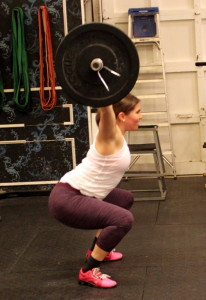## Saturday, January 3, 2015

### Saturday, January 3, 2015CrossFit Total
back squat, 1 repetition
shoulder press, 1 repetition

This workout is scored by the total weight lifted.

Compare results to October 14, 2013.

### 1 Comment

1.–Scores–
Ryan L.: 250*+135*+290* = 675-lbs.
Sean F.: 245*+135*+375* = 755-lbs.
James H.: 215+140*+255 = 610-lbs.
Jim D.: 205+125*+250 = 580-lbs.
Kyle S.: 215*+122*+300* = 637-lbs.
John L.: 130*+340* = 470-lbs. (sub: 2′ plank hold + 25x strict sit-up; 5 rounds)
Dave B.: 205*+120*+225* = 550-lbs.
Rob C.: 190*+145*+295* = 630-lbs.
Shawn J.: 190+95+260* = 545-lbs.
Krishnan S.: 205*+102+260 = 567-lbs.
David S.: 250+135*+330 = 715-lbs.
Josh M.: 240+95+310* = 645-lbs.
Steve M.: 230+130+255 = 615-lb.
Jon H.: 250+115+295 = 660-lbs.
Holmes H.: 145+80+165 = 390-lbs.
Katie K.: 170*+77*+195* = 442-lbs.
Lisa C.: 135+80+205* = 420-lbs.
Buffy M.: 110*+77*+165* = 352-lbs.
Dat D.: 335*+135*+360* = 830-lbs.
Melody F.: 245+100*+275 = 620-lbs.
John G.: 300*+180+425= 905-lbs.
Walt C.: 185*+120*+245* = 550-lbs.
Neil A.: 165+85+245 = 495-lbs. (sub: back squat; 3RM, shoulder press; 3RM, deadlift; 3RM)
Diane N.: 125*+75*+155* = 355-lbs.
Ran B.: 95*+100*+180* = 375-lbs. (sub: back squat; 3RM, shoulder press; 3RM)
Joanna T.: 160+80*+210* = 450-lbs.
Alissa G.: 105+75+185 = 365-lbs. (sub: back squat; 3RM, shoulder press; 3RM, deadlift; 3RM)
Christine S.: 135+90+85 = 410-lbs.
Alexis P.: 155*+65+165* = 385-lbs. (sub: shoulder press; 3RM)
Kevin K.: 115+85*+205* = 405-lbs. (sub: front squat; 3RM, shoulder press; 3RM, deadlift; 2RM)
Tracey S.: 115*+75*x+205* = 395-lbs. (sub: back squat; 3RM, shoulder press; 3RM, deadlift; 2RM)
David G.: 115*+85*+185* = 385-lbs. (sub: back squat; 3RM, shoulder press; 3RM, deadlift; 2RM)
Jamie T.: 275*+155+400* = 830-lbs.
Joe T.: 265*+135*+325* = 725-lbs.
Matt H.: 225*+120+350 = 695-lbs.
Chris T.: 240*+135*+305* = 680-lbs.
Teal B.: 165+95*+95 = 355-lbs. (sub: back squat; 5RM)
Rebekka E.: 135+95+235 = 465-lbs.
Mayra C.: 160+80+225 = 465-lbs.
Donal O.: 285+120+380* = 785-lbs.